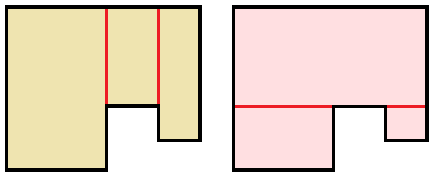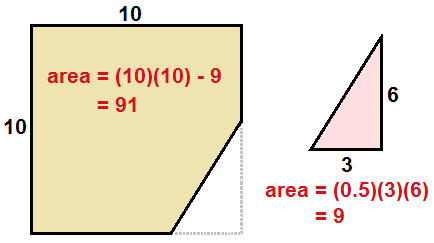# How to Calculate Your Home's Square Footage

There are many cases where it is important to know the exact square footage of your home, such as a home appraisal or sale. If you underestimate the square footage, you risk losing buyers who want a larger house. If you overestimate the area of your house, you may be accused of misrepresenting your home's value.

To accurately calculate the square footage of your home, no matter its shape, use the following guide.

First, get a pad of graph paper that has at least 30 rows and 30 columns of squares. Let each square on the graph represent a 2-by-2 foot square. This will give you enough space to sketch the floor plan of a house that is at most 60 feet by 60 feet. If your house is larger, you can make the scale smaller.

Next, measure the lengths of the outside edges of your house, and sketch it on the graph paper. Keep measurements as accurate as possible. If you need to convert inches to feet, use 3" = 0.25', 4" = 0.333', and 6" = 0.5'. For example, if one side is 20' 6", this is equivalent to 20.5'.

After sketching the perimeter of your house, divide the area into smaller rectangles. (This is unnecessary if your house is a perfect rectangle or square.) There is more than one correct way to partition the square footage into rectangles, so you have some freedom in choosing how to simplify the area. See the image below:Compute the area of every rectangle, then add them to find the total square footage of your home's first level. If the second floor completely covers the first floor, multiply by 2 to get the total area of both levels.

For parts of the house that are in the shape of circular arcs, remember that the area of a full circle is (pi)r2. The area of a half circle is (1/2)(pi)r2, a quarter circle is (1/4)(pi)r2, and three quarters of a circle is (3/4)(pi)r2.

For parts of the home that are shaped like triangles or "missing triangles," recall that the area of a triangle is 1/2 times base times height.For most real estate purposes, you can only include finished parts of your home in the square footage calculation. Any unfinished attic or basement spaces should not be counted, unless your state or county has different regulations.